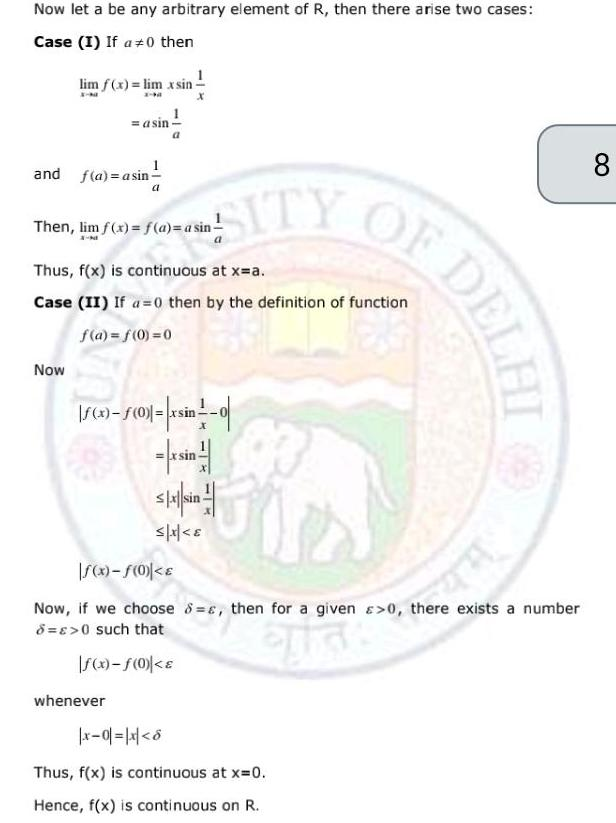Question:

# Now let a be any arbitrary element of R then there arise two

Last updated: 9/13/2023Now let a be any arbitrary element of R then there arise two cases Case I If a 0 then lim f x lim xsin X N and f a asin asin Now Then lim f x f a a sin connu DELI lin 0 a ITY Thus f x is at x a Case II If a 0 then by the definition of function f a f 0 0 x f 0 xsin 0 Skx sin x whenever Fir f x f 0 E Now if we choose 8 s then for a given 0 there exists a number 8 0 such that f x f 0 x 0 x 6 Thus f x is continuous at x 0 Hence f x is continuous on R 8